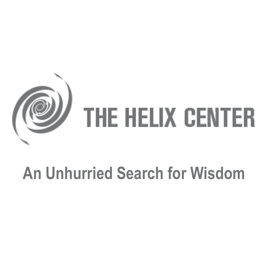1h 48 min# Mechanization of Math The Helix Center

• Ciências naturais

Proof, in the form of step by step deduction, following the rules of logical reasoning, is the ultimate test of validity in mathematics. Some proofs, however, are so long or complex, or both, that they cannot be checked for errors by human experts. In response, a small but growing community of mathematicians, collaborating with computer scientists, have designed systems that allow proofs to be verified by machine. The success in certifying proofs of some prestigious theorems has led some mathematicians to propose a complete rethinking of the profession, requiring future proofs to be written in computer readable code. A few mathematicians have gone so far as to predict that artificial intelligence will replace humans in mathematical research, as in so many other activities.
One’s position on the possible future mechanization of proof is a function of one’s view of mathematics itself. Is it a means to an end that can be achieved as well, or better, by a competent machine as by a human being? If so, what is that end, and why are machines seen as more reliable than humans? Or is mathematics rather an end in itself, a human practice that is pursued for its intrinsic value? If so, what could that value be, and can it ever be shared with machines?

Proof, in the form of step by step deduction, following the rules of logical reasoning, is the ultimate test of validity in mathematics. Some proofs, however, are so long or complex, or both, that they cannot be checked for errors by human experts. In response, a small but growing community of mathematicians, collaborating with computer scientists, have designed systems that allow proofs to be verified by machine. The success in certifying proofs of some prestigious theorems has led some mathematicians to propose a complete rethinking of the profession, requiring future proofs to be written in computer readable code. A few mathematicians have gone so far as to predict that artificial intelligence will replace humans in mathematical research, as in so many other activities.
One’s position on the possible future mechanization of proof is a function of one’s view of mathematics itself. Is it a means to an end that can be achieved as well, or better, by a competent machine as by a human being? If so, what is that end, and why are machines seen as more reliable than humans? Or is mathematics rather an end in itself, a human practice that is pursued for its intrinsic value? If so, what could that value be, and can it ever be shared with machines?

1h 48 min